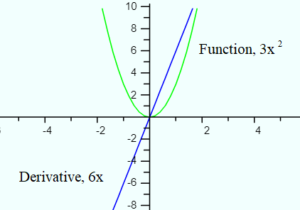# 4 Concepts of Calculus

## What are the 4 Concepts of Calculus?

The four central concepts of calculus are (Ledder, 2020):

These four concepts are the foundation for all of the material in calculus. They allow you to solve mathematical problems that were, prior to the discovery of calculus, impossible to solve.

## Continuity and Limits

Continuity is very difficult to define precisely, but in layman’s terms is fairly easy to visualize: It means that something goes on and on forever, without any breaks.One of the 4 concepts of calculus is continuity. The atomic clock measure time-continuity.The simplest example of continuity is time. Time is never ending (well, you could argue it began with the Big Bang, but let’s not argue the minutiae!). If you try and count from the beginning of time until the end of time, well… you can’t. It’s also continuous if you zoom in to a particular point (or try to). Let’s say is takes you 20 seconds to run 100m. It’s probably not precisely 20 seconds; More likely it’s 20.01 seconds, 20.001 seconds, or even 20.00000001 seconds. The most accurate measuring device we have for time is the atomic clock, swimming at 500 trillion times per second and reports time with 19 decimal places. But that doesn’t mean time only exists in 19 decimal place increments; It’s just the best we can measure—for now.

Calculus deals with continuous functions using the limit process, which you can think of as a “stopping point”. In the atomic clock example, the limit of the clock’s accuracy is 19 decimal places. Limits are in place to give us a workable number, otherwise we would be calculating (with ordinary mathematics) forever and ever.

## Definite Integral and DerivativeThe derivative gives you the slope of a function.The remaining two concepts are usually defined as solutions to geometry problems. For example, the derivative is the slope at a point, and the definite integral is the area under a curve. Theses two core concepts allow you to take a simple graph, like one of the velocity of a car, and mine it for information you couldn’t get with ordinary mathematics. For example, you can find acceleration or “jerk“—that feeling you get in an elevator when it suddenly slows down or speeds up.

## References

Ledder, G. (2020). A Brief Summary of Calculus. Retrieved November 11, 2020 from: http://www.math.unl.edu/~gledder1/Math238/Calculus

CITE THIS AS:
Stephanie Glen. "4 Concepts of Calculus" From StatisticsHowTo.com: Elementary Statistics for the rest of us! https://www.statisticshowto.com/4-concepts-of-calculus/